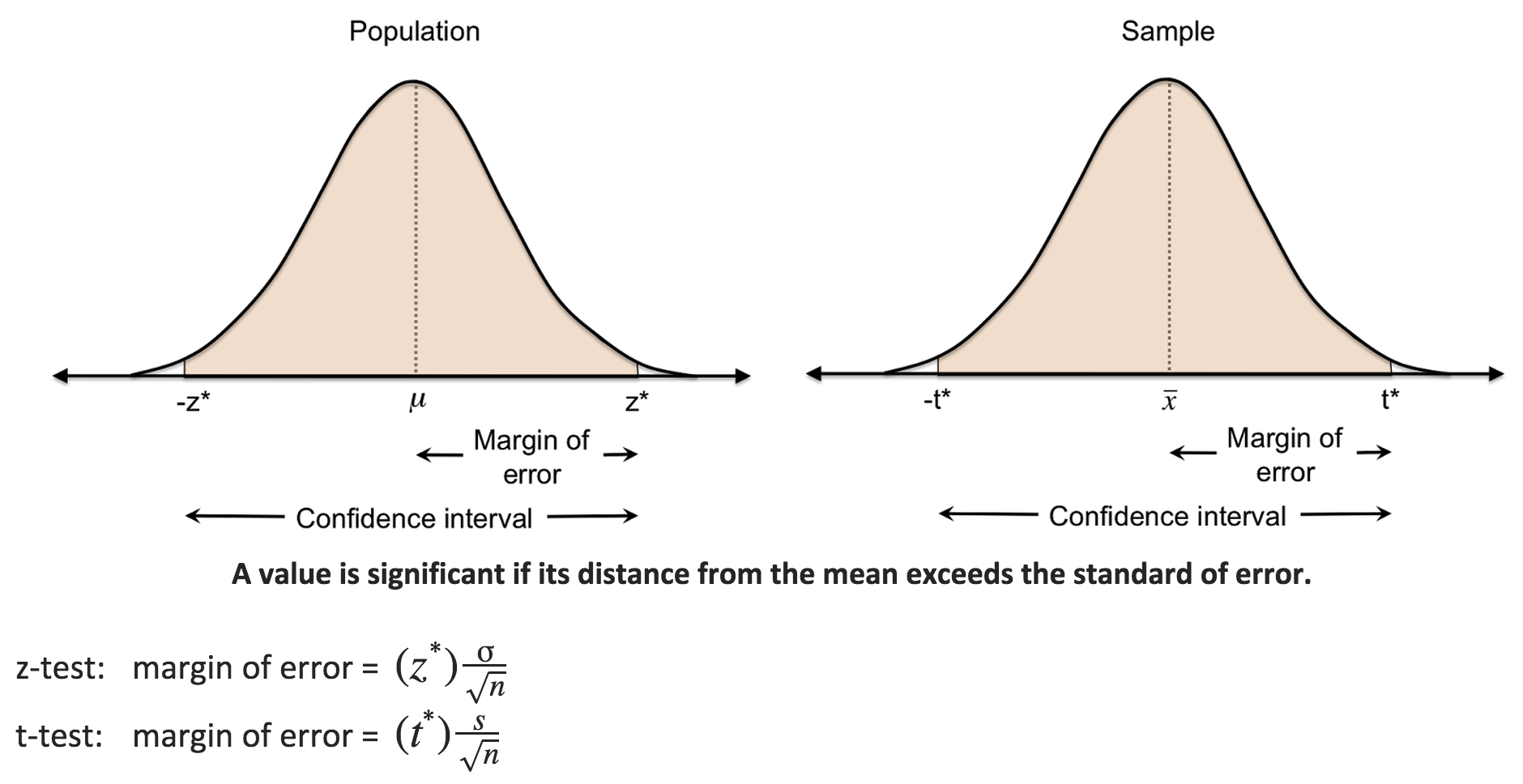PURCHASE A DIGITAL COPY PURCHASE A HARD COPY Lesson 1 Introduction to Statistical Research Methods Lesson 2 Visualizing Data Lesson 3 Central Tendency Lesson 4 Variability Lesson 5 Standardizing Lesson 6 Normal Distribution Lesson 7 Sampling Distributions Lesson 8 Estimation Lesson 9 Hypothesis Testing Lesson 10 t-Tests for Dependent Samples Lesson 11 t-Tests for Independent Samples Lesson 12 Intro to One-Way ANOVA Lesson 13 One-Way ANOVA: Test significance of differences Lesson 14 Correlation Lesson 15 Linear Regression Lesson 16 Chi-Squared Tests Afterward Index

Now that you know what the F-statistic is and how to find it, we can do a “multiple-comparison test” to determine which populations are significantly different. The most common multiple-comparison test involves computing Tukey’s HSD (honestly significant difference). If the difference between two means are greater than Tukey’s HSD, they are considered honestly significantly different. (Yes, kinda silly.)

Tukey’s HSD is just like the margin of error, which if you remember is the critical value (z* or t*) times the standard error (i.e., the number of standard errors from the mean). Recall that the margin of error is half the length of the confidence interval.With CIs, if the distance between a value and the mean is greater than the margin of error, the difference is considered statistically significant. With this multiple-comparison test for ANOVA, if the distance between any two means exceeds Tukey’s HSD, the difference is considered statistically significant.

To continue learning, purchase Street-Smart Stats: A Friendly Introduction to Statistical Research Methods.